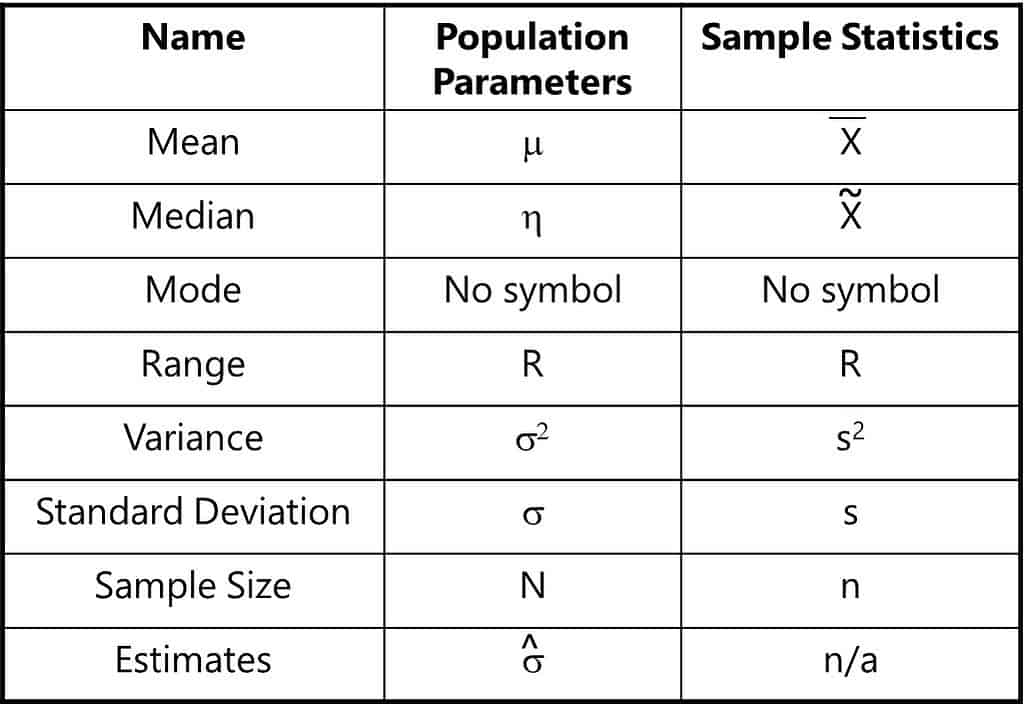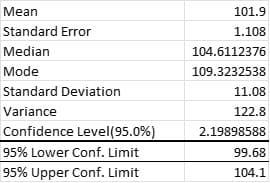Statistics is a branch of math that involves the collection, description, analysis, and inference of conclusions from quantitative data. But what is a statistic? Let’s find out.

The word statistic is a word with numerous possible definitions. In its simplest form, a statistic can be defined as a descriptor of a set of data. The data you collect can be defined as either population data or sample data. The descriptors of data include its central tendency and dispersion or variation.

## Overview: What is a statistic?

Strictly speaking, you can define the characteristics of population data or sample data. The descriptors of population data are referred to as population parameters. When it is not feasible to directly measure the value of a population parameter, statistical methods are used to infer the likely value of the parameter based on a statistic computed from a sample taken from the population.

The descriptor of sample data is referred to as a sample statistic. When a sample statistic is used for estimating a population parameter, the statistic is called an estimator. A descriptive statistic is used to summarize sample data. A test statistic is used in statistical hypothesis testing

Here is a table describing both population parameters and sample statistics and their relevant symbols. Note that population parameter symbols are generally Greek letters.## An industry example of a statistic

The company’s statistician did some analysis and descriptive statistics of company sample data shown below.He was able to use the sample statistics to estimate the population parameter for the mean. By adding and subtracting the value for the Confidence Level (2.199) he computed a confidence interval or 99.68 – 104.1 as the estimate of the mean of the population parameter.

### What characteristics of data do sample statistics describe?

Sample statistics are used to describe the central tendency (mean, median, and mode) as well as the variation of the data (range, variance, and standard deviation).

### Can I use a sample statistic to estimate a population parameter?

Yes, but understand you will have some error since you are using a subset of the larger data. In many cases, it is not possible to collect all the population data, so you have no choice but to use an estimate.

### What’s the difference between statistics and a statistic?

A statistic is the descriptor of a set of sample data. Statistics is the broader concept of the process of designing, comparing, interpreting, and analyzing data. Statistics is concerned with the sample data and not the population data since the population data is almost impossible to collect.